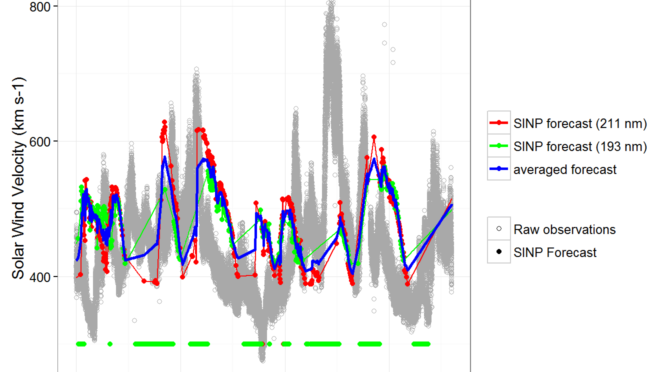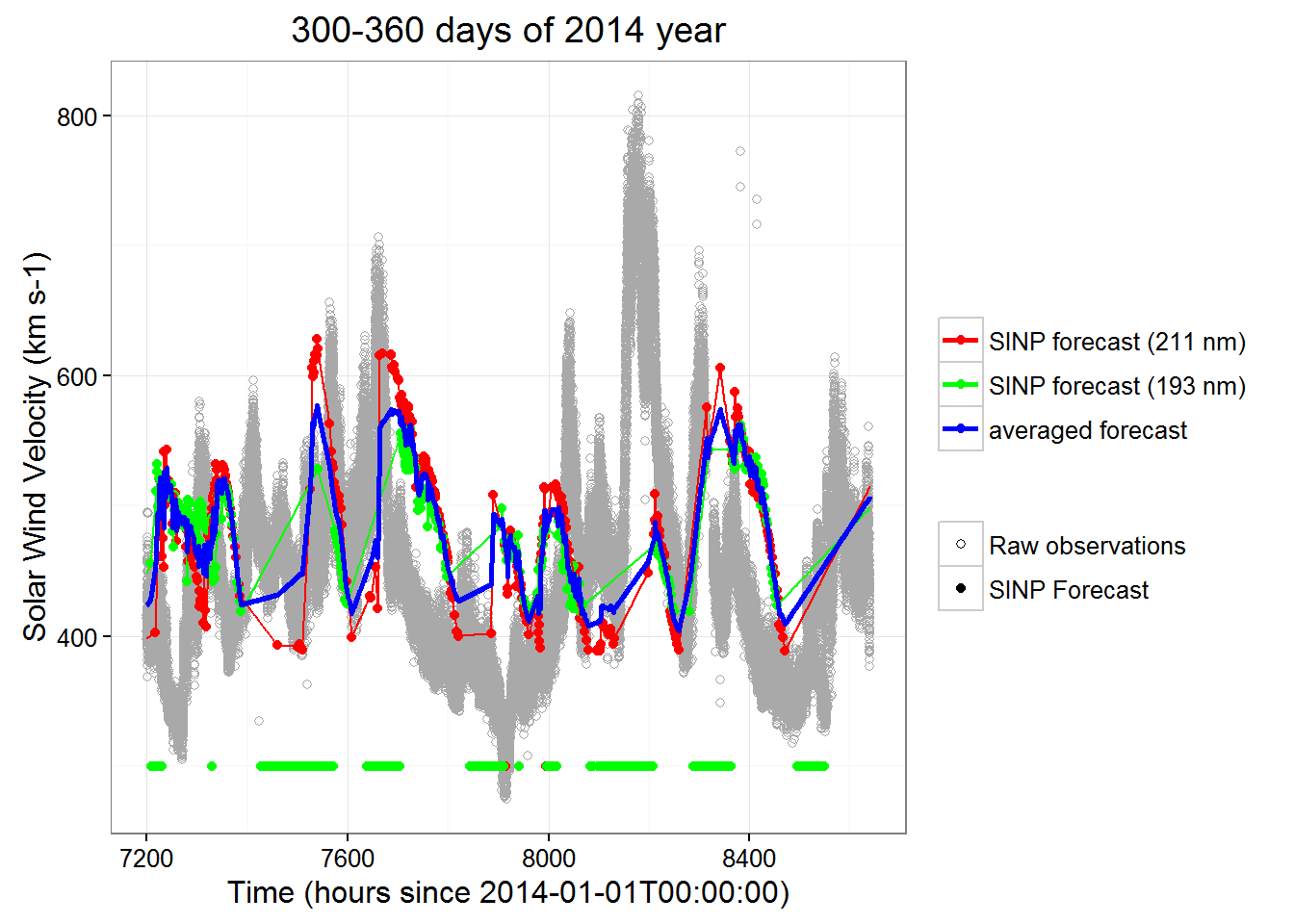# MSU SINP Solar Wind Velocity ForecastThis is a part of study described in the dedicated post.

We will compare “out-of-sample” error of MSU SINP model, Wind Pulses simulator and machine learning regression model. In this post we will assess current SINP solar wind predictions.

We need to define error measure for models comparison.
As we predict quantitative value of solar wind velocity, the good error measure could be RMSE. We will also calculate R2 for each of the predictions to analyse how much variance is described by a model.

Our testing data set will be days 300 – 360 of year 2014. We will use this interval to evaluate prediction error rates for every model.

SINP predictions contain lots of predicted values equal to 300. Their presence produce significant oscillations between value of 300 and other higher values (see figure below). Such oscillations can’t happen in real life. The simplest way to eliminate these oscillations is to filter out predictions equal to 300. These points with value 300 will not be accounted during interpolation of predictions.RMSE of SINP model is 93.3.

RMSE of null model is 93.4.

R2 of SINP model is 0.0025192.

(null model here is average observed wind speed velocity over entire 2014 year)

Complete R report is available here.